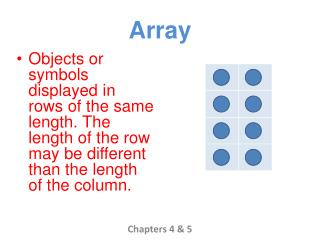DownloadDownload PresentationArray

# Array

Download Presentation## Array

- - - - - - - - - - - - - - - - - - - - - - - - - - - E N D - - - - - - - - - - - - - - - - - - - - - - - - - - -
##### Presentation Transcript

1. Array • Objects or symbols displayed in rows of the same length. The length of the row may be different than the length of the column. Chapters 4 & 5

2. Communicative Property of Multiplication • The order in which two numbers are multiplied does not change the product. 2 X 4 = 8 4 X 2 = 8 2 X 4 = 4 X 2 Chapters 4 & 5

3. Factor 2 X 4 = 8 • A number that is multiplied by another number. • A number that divides into a whole number evenly. 8 4 =2 Chapters 4 & 5

4. Identity Property of Multiplication • If you multiply a number by 1, the product is the same as the given number. 2 X 1 = 2 Chapters 4 & 5

5. Multiply 2 X 4 = 8 Two groups of four is equal to the product eight. Also known as repeated addition. 2 + 2 + 2 + 2= 8 • Find the product Chapters 4 & 5

6. Product • The answer to a multiplication problem. 2 X 4 = 8 Chapters 4 & 5

7. Zero Property of Multiplication • Any number multiplied by zero is zero. 2 X 0 = 0 Chapters 4 & 5

8. Associative Property of Multiplication • The grouping of the factors does not change the product. (3 X 2) X 6 = 36 3 X (6X 2) = 36 Chapters 4 & 5

9. Rule Rule: Multiply by 3 • Tells you what to do to the first number to get the second number. Chapters 4 & 5